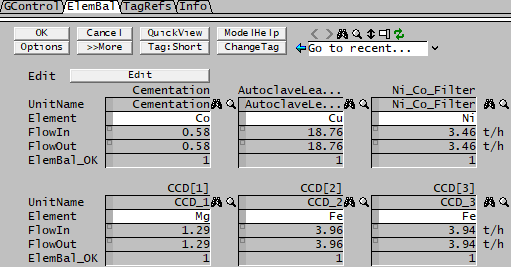# Example Class - Check Elemental Balance of a Process Unit Operation

Navigation: PGMs ➔ Example PGM Files ➔ Check Elemental Balance

SysCAD Pre-Defined Classes and Examples EXAMPLES: User Defined Classes
Sp Databse
Class
PSD
Class
Array
Class
StrArray
Class
Matrix
Class
Tag Select
Class
Plant Model
Class
Noise
Class
Time
Class
Agitator
Power
Reaction
Finder
Aq Feed Conc
Calculator
Evaporation
Correlation
Bayer Liquor
Class
Check
Element Bal

 ```; ---- Define the CLASS and its Functions ---- Class UnitElemMB TextLabel() String UnitName{Tag} String Element*{Comment("If unspecified, defalut to 'O' for Oxygen")} String Stream, FlowTag Real [email protected]("Qm", "kg/h"), [email protected]("Qm", "kg/h") Real [email protected]@("Qm", "kg/h"), TotFlow integer Count, [email protected]@, [email protected]@, i Checkbox [email protected] StrFunction GetStreamName(integer i, String InOrOut) if IsEmpty(Element) element = "O" Endif return [Concatenate(UnitName,".Links.",InOrOut,".[",IntToStr(i),"].Flange")] EndFunct Function GetFlow(String InOrOut) Count = [Concatenate(UnitName,".Links.",InOrOut,".Count")] TotFlow = 0.0 i = 0 while (i < Count) Stream = GetStreamName(i,InOrOut) FlowTag = Concatenate(Stream,".Qo.QEl.",Element," (kg/h)") TotFlow = TotFlow + [FlowTag] i = i + 1 EndWhile return TotFlow EndFunct Sub CalculateBalance() FlowIn = GetFlow("In") inCount = Count FlowOut = GetFlow("Out") outCount = Count MassDiff = FlowOut - FlowIn ElemBal_OK = iif(Abs(MassDiff) < 0.00001, 1, 0) EndSub EndClass ; ---- Using the Class ---- PageLabel(ElemBal) ; Define the required number of Elemental Balance Calculations UnitElemMB Element_balance integer j ; Perform the Elemental Balance Calculations at the end of project solve Sub TerminateSolution() j = 0 while (j < 6) Element_balance[j].CalculateBalance() j = j + 1 EndWhile EndSub \$ ``` Example General Controller results for the Nickel Copper Demo Project.